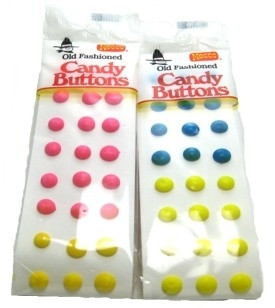Saturday, January 2, 2016

Button Candies To Teach an Understanding of the Meaning of Multiplication

I've been working with a 3rd Grader to develop an understanding of the meanings of multiplication and division of whole numbers through activities and problems involving equal-sized groups, arrays, and area model.

Today we created these flashcards together that illustrate the meaning of the equation.

I like to think of the equation as a sentence.
The sentence says, " Three groups of seven are the same as twenty-one."

When you read the equation in this way, it starts making sense... not just a multiplication fact that you need to memorize.

Notice that we added more meaning by showing the relationship to repeated addition on the left-hand side of the card.We used this candies to create the arrays.  The 3rd Grader said that it was the yummiest math lesson ever!

When we created the array for the equation
3 X 0 = 0
I thought the final model really showed the concept:
We peeled the candy buttons and left a residue that showed
a value of nothing.
Interestingly, when I gave this student a screening test for her multiplication concepts, it showed that she did not understand the concept of multiplying a factor by zero.
Now I feel that she does understand

The Multiplication Property of Zero

Future lessons will use the cards that we created.

Deborah